Question

Researchers believe that 24.5% of the adults in the United States are obese. A Gallup poll examined the rate of obesity in America with a survey 86,664 randomly sampled U.S. adults. Of the adults surveyed, 23,053 said that they were obese. Is this enough evidence to state that more than 24.5% of all adults in the U.S. are obese

1.Latifah

The p-value of the test is 0 < 0.05(standard significance level), which means that this is enough evidence to state that more than 24.5% of all adults in the U.S. are obese

Step-by-step explanation:

Researchers believe that 24.5% of the adults in the United States are obese. Test if there is enough evidence to state that more than 24.5% of all adults in the U.S. are obese.

At the null hypothesis, we test if the proportion is of 24.5% or less, that is: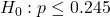At the alternative hypothesis, we test if this proportion is greater than 24.5%, that is: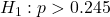The test statistic is: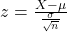In which X is the sample mean,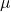is the value tested at the null hypothesis,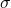is the standard deviation and n is the size of the sample.

0.245 is tested at the null hypothesis:

This means that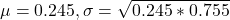Survey 86,664 randomly sampled U.S. adults. Of the adults surveyed, 23,053 said that they were obese.

This means that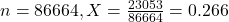Value of the test statistic: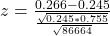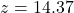P-value of the test and decision:

The p-value of the test is the probability of finding a sample proportion of 0.266 or higher, which is 1 subtracted by the p-value of z = 14.37.

The p-value of z = 14.37 is 1.

1 – 1 = 0

The p-value of the test is 0 < 0.05(standard significance level), which means that this is enough evidence to state that more than 24.5% of all adults in the U.S. are obese